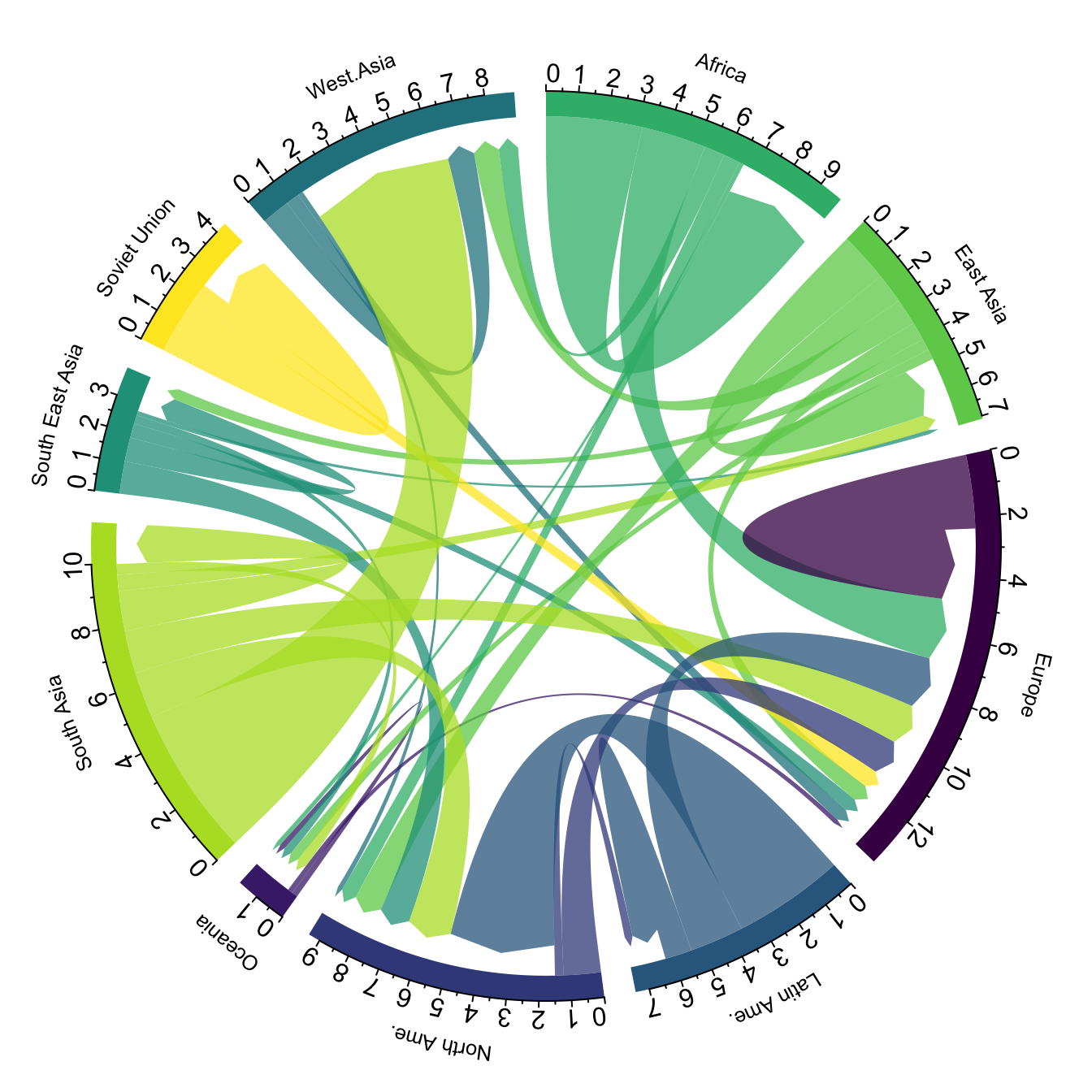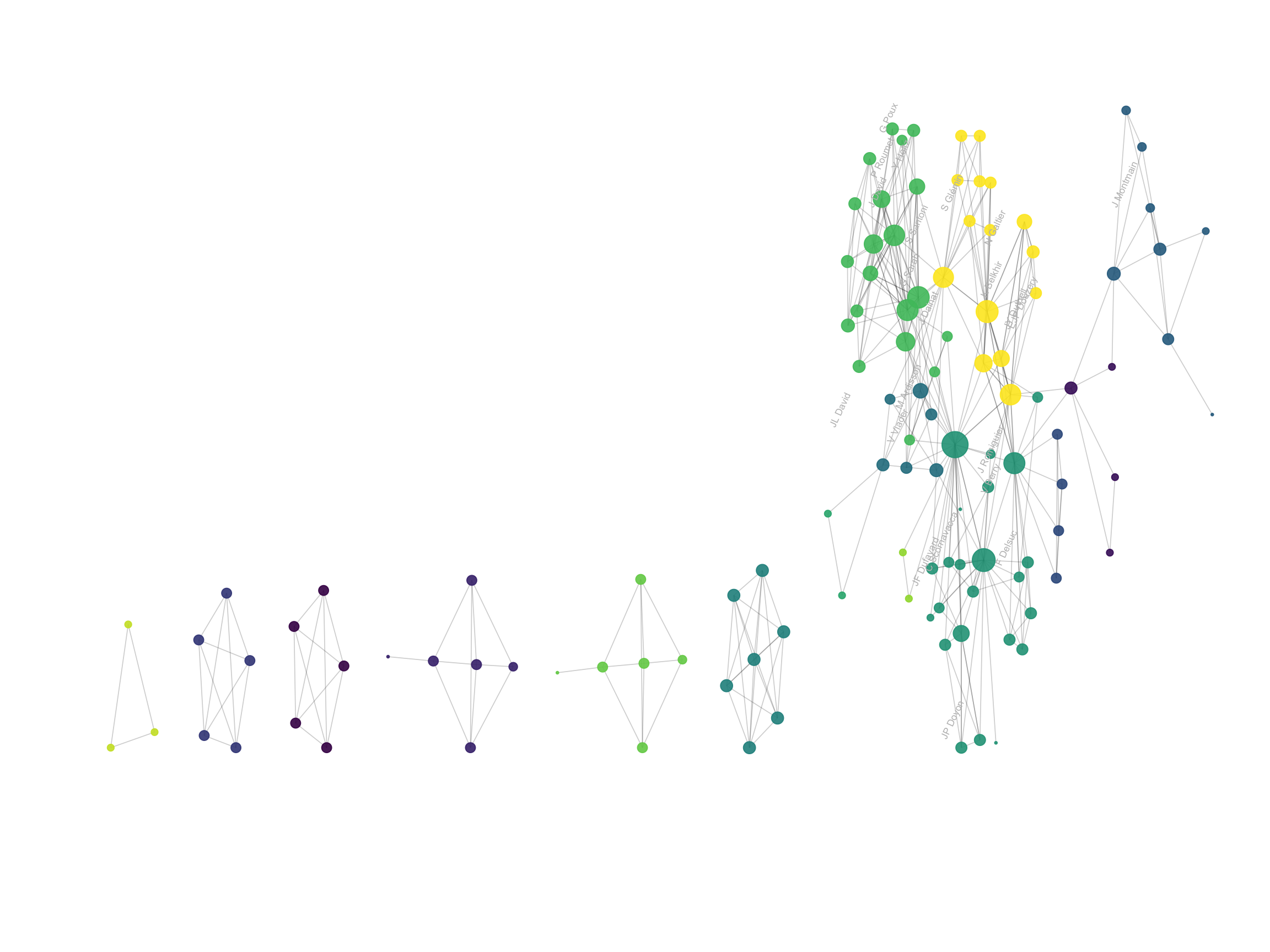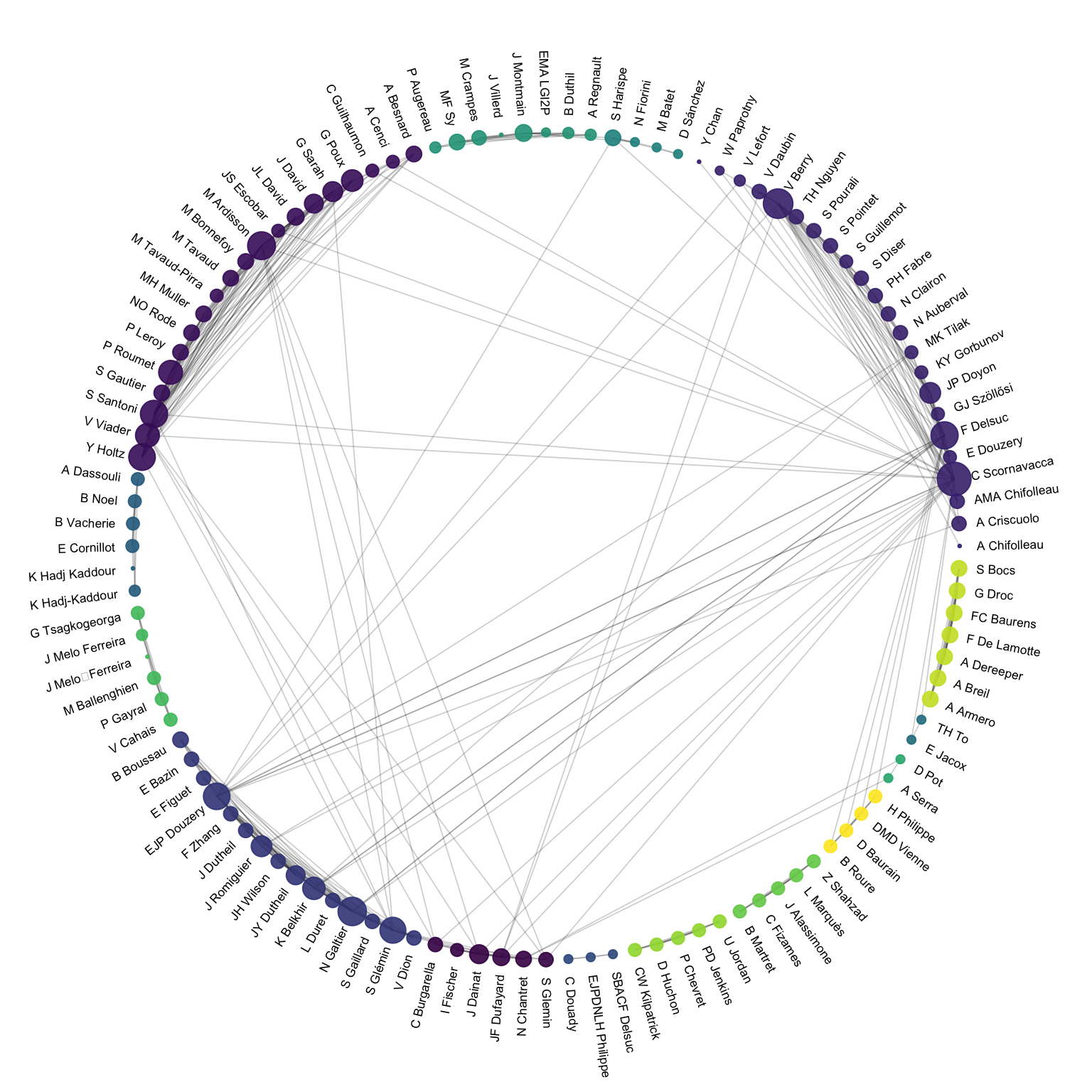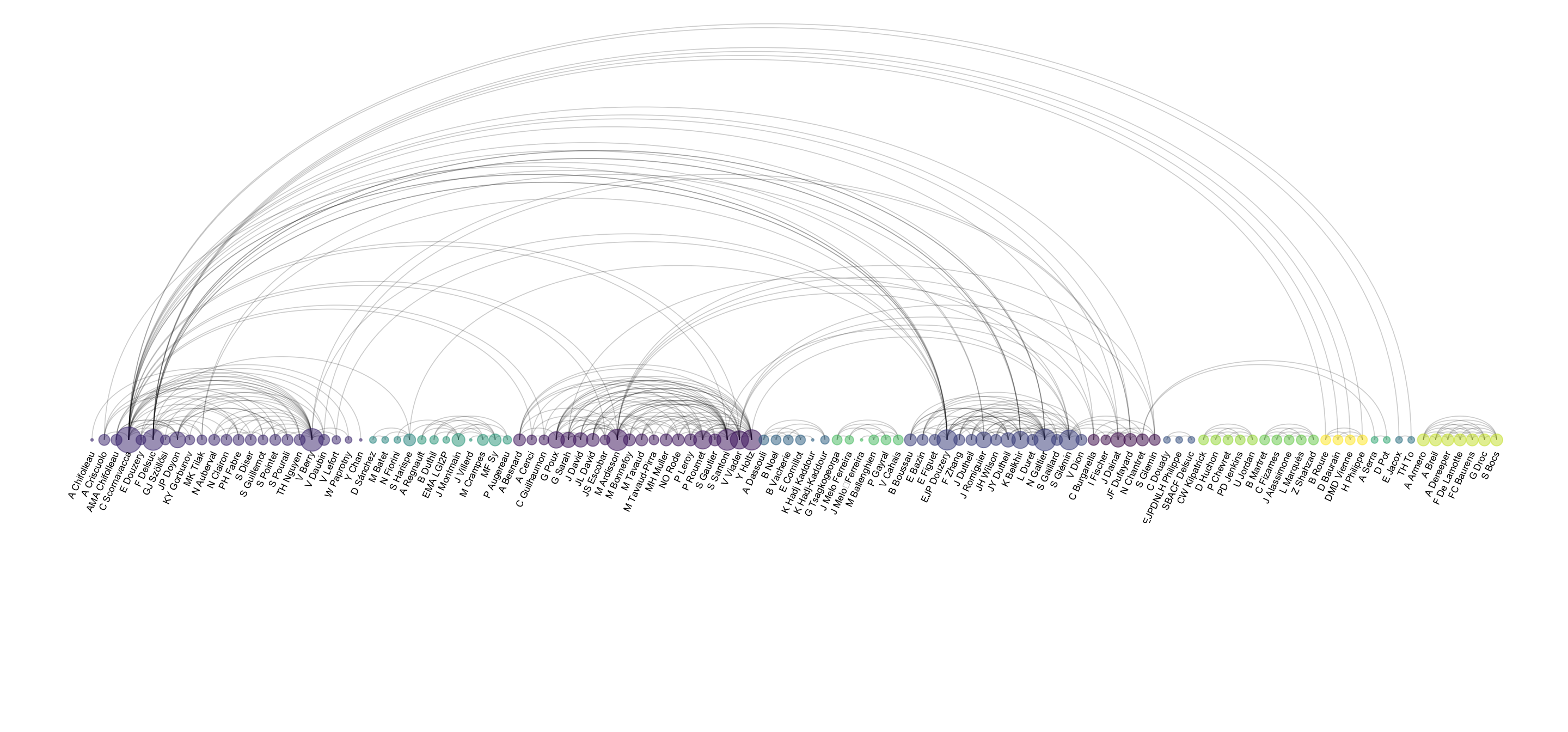Adjacency and incidence matrix provide relationship between several nodes. The information they contain can have different nature, thus this document will consider several examples:

• Relationships can be `directed` and `weighted`. Like the number of people migrating from one country to another. Data used comes from this scientific publication from Gui J. Abel.
``````# Libraries
library(tidyverse)
library(hrbrthemes)
library(circlize)
library(kableExtra)
options(knitr.table.format = "html")
library(viridis)
library(igraph)
library(ggraph)
library(colormap)

# show data
data %>% head(3) %>% select(1:3) %>% kable() %>%
kable_styling(bootstrap_options = "striped", full_width = F)``````
Africa East.Asia Europe
Africa 3.142471 0.000000 2.107883
East Asia 0.000000 1.630997 0.601265
Europe 0.000000 0.000000 2.401476
• Relationships can be `undirected` and `unweighted`. I will consider all the co-authors of a researcher and study who is connected through a common publication. Data have been retrieved using the scholar package, the pipeline is describe in this github repository. The result is an adjacency matrix with about 100 researchers, filled with 1 if they have published a paper together, 0 otherwise.
``````# Load data

# show data
dataUU %>% head(3) %>% select(1:4) %>% kable() %>%
kable_styling(bootstrap_options = "striped", full_width = F)``````
from A.Bateman A.Besnard A.Breil
A Armero NA NA 1
A Bateman NA NA NA
A Besnard NA NA NA
• Relationships can also be `undirected` and `weighted`

• Relationships can also be `directed` and `unweighted`

# Chord diagram

Chord diagram is a good way to represent the migration flows. It works well if your data are directed and weighted like for migration flows between country.

Disclaimer: this plot is made using the circlize library, and very strongly inspired from the Migest package from Gui J. Abel, who is also the author of the migration dataset used here.

Since this kind of graphic is used to display flows, it can be applied only on network where connection are `weighted`. It does not work for the other example on authors connections.

``````# short names
colnames(data) <- c("Africa", "East Asia", "Europe", "Latin Ame.",   "North Ame.",   "Oceania", "South Asia", "South East Asia", "Soviet Union", "West.Asia")
rownames(data) <- colnames(data)

# I need a long format
data_long <- data %>%
rownames_to_column %>%
gather(key = 'key', value = 'value', -rowname)

# parameters
circos.clear()
circos.par(start.degree = 90, gap.degree = 4, track.margin = c(-0.1, 0.1), points.overflow.warning = FALSE)
par(mar = rep(0, 4))

# color palette
mycolor <- viridis(10, alpha = 1, begin = 0, end = 1, option = "D")
mycolor <- mycolor[sample(1:10)]

# Base plot
chordDiagram(
x = data_long,
grid.col = mycolor,
transparency = 0.25,
directional = 1,
direction.type = c("arrows", "diffHeight"),
diffHeight  = -0.04,
annotationTrack = "grid",
annotationTrackHeight = c(0.05, 0.1),

circos.trackPlotRegion(
track.index = 1,
bg.border = NA,
panel.fun = function(x, y) {

xlim = get.cell.meta.data("xlim")
sector.index = get.cell.meta.data("sector.index")

# Add names to the sector.
circos.text(
x = mean(xlim),
y = 3.2,
labels = sector.index,
facing = "bending",
cex = 0.8
)

circos.axis(
h = "top",
major.at = seq(from = 0, to = xlim, by = ifelse(test = xlim>10, yes = 2, no = 1)),
minor.ticks = 1,
major.tick.percentage = 0.5,
labels.niceFacing = FALSE)
}
)``````In my opinion this is a powerful way to display information. Major flows are easy to detect, like the migration from South Asia towars Westa Asia, or Africa to Europe. Moreover, for each continent it is quite easy to quantify the proportion of people leaving and arriving.

However chord diagram is not an usual way of displaying information. Thus, it is advised to give a good amount of explanation to educate your audience. A good way to do so is to draw just a few connections in a first step, before displaying the whole graphic. See this blog post by Nadieh Bremer for more ideas on this topic.

# Sankey diagram

Sankey diagram is another option to display weighted connection. Instead of displaying regions on a circle, they are duplicated and represented on both side of the graphic. Origin is usually on the left, destination on the right.

``````# Package
library(networkD3)

# I need a long format
data_long <- data %>%
rownames_to_column %>%
gather(key = 'key', value = 'value', -rowname) %>%
filter(value > 0)
colnames(data_long) <- c("source", "target", "value")
data_long\$target <- paste(data_long\$target, " ", sep="")

# From these flows we need to create a node data frame: it lists every entities involved in the flow
nodes <- data.frame(name=c(as.character(data_long\$source), as.character(data_long\$target)) %>% unique())

# With networkD3, connection must be provided using id, not using real name like in the links dataframe.. So we need to reformat it.
data_long\$IDsource=match(data_long\$source, nodes\$name)-1
data_long\$IDtarget=match(data_long\$target, nodes\$name)-1

# prepare colour scale
ColourScal ='d3.scaleOrdinal() .range(["#FDE725FF","#B4DE2CFF","#6DCD59FF","#35B779FF","#1F9E89FF","#26828EFF","#31688EFF","#3E4A89FF","#482878FF","#440154FF"])'

# Make the Network
sankeyNetwork(Links = data_long, Nodes = nodes,
Source = "IDsource", Target = "IDtarget",
Value = "value", NodeID = "name",

# Heatmap

The heatmap is another great alternative to represent an adjacency matrix. Here, all the origin countries are represented as row, and all the destination as columns. The diagonal pops out with a lot of yellow squares, what means that most of the migrations are intra continental.

``````library(heatmaply)
p <- heatmaply(data,
dendrogram = "none",
xlab = "", ylab = "",
main = "",
scale = "column",
margins = c(60,100,40,20),
grid_color = "white",
grid_width = 0.00001,
titleX = FALSE,
hide_colorbar = TRUE,
branches_lwd = 0.1,
label_names = c("From", "To:", "Value"),
fontsize_row = 7, fontsize_col = 7,
labCol = colnames(data),
labRow = rownames(data),
heatmap_layers = theme(axis.line=element_blank())
)``````

Note that if the matrix is `unweighted`, each connection can have only 2 values: 1 if there is a connection, 0 otherwise. It is the case for the co-authorship network example, where researchers are connected if they have already published a paper together. The heatmap below shows these connection and also apply a clustering algorithm to the data: researchers that tend to be involved in the same papers are grouped together.

``````# Format data
tmp <- dataUU
rownames(tmp) <- tmp\$from
tmp <- tmp %>% select(-from)
tmp[is.na(tmp)] <- 0

# Keep people with more than 1 connections
tmp <- tmp[which(rowSums(tmp)>3), which(colSums(tmp)>3)]

# Heatmap
p <- heatmaply(tmp,
dendrogram = "both",
xlab = "", ylab = "",
main = "",
scale = "none",
margins = c(60,100,40,20),
grid_color = "white",
grid_width = 0.0000000001,
titleX = FALSE,
hide_colorbar = TRUE,
branches_lwd = 0.1,
label_names = c("Name", "With:", "Value"),
fontsize_row = 7, fontsize_col = 7,
labCol = colnames(tmp),
labRow = rownames(tmp),
heatmap_layers = theme(axis.line=element_blank())
)``````

# Network

Since an adjacency matrix is a `network structure`, it is possible to build a network graph. In a network graph, each entity is represented as a `node`, and each connection as an `edge`.

In my opinion, this type of representation makes more sense when the connection are `unweighted`, since drawing edges with different sizes tends to clutter the figure and make it unreadable.

Thus, here is an application of this chart type to the coauthor network. Researchers are the nodes, represented as dots. If 2 researchers have published at least one scientific paper together, they are connected. The node size is proportionnal to the number of coauthors.

``````# Transform the adjacency matrix in a long format
connect <- dataUU %>%
gather(key="to", value="value", -1) %>%
mutate(to = gsub("\\.", " ",to)) %>%
na.omit()

# Number of connection per person
c( as.character(connect\$from), as.character(connect\$to)) %>%
as.tibble() %>%
group_by(value) %>%
summarize(n=n()) -> coauth
colnames(coauth) <- c("name", "n")

# Create a graph object with igraph
mygraph <- graph_from_data_frame( connect, vertices = coauth, directed = FALSE )

# Find community
com <- walktrap.community(mygraph)

#Reorder dataset and make the graph
coauth <- coauth %>%
mutate( grp = com\$membership) %>%
arrange(grp) %>%
mutate(name=factor(name, name))

# keep only 10 first communities
coauth <- coauth %>%
filter(grp<16)

# keep only this people in edges
connect <- connect %>%
filter(from %in% coauth\$name) %>%
filter(to %in% coauth\$name)

# Create a graph object with igraph
mygraph <- graph_from_data_frame( connect, vertices = coauth, directed = FALSE )

# prepare a vector of n color in the viridis scale
mycolor <- sample(mycolor, length(mycolor))

# Make the graph
ggraph(mygraph) +
geom_node_point(aes(size=n, color=as.factor(grp), fill=grp), alpha=0.9) +
scale_size_continuous(range=c(0.5,8)) +
scale_color_manual(values=mycolor) +
geom_node_text(aes(label=ifelse(n>6, as.character(name), "")), angle=65, hjust=rep(c(0,1),58), nudge_y = rep(c(0.5,-0.5),58), size=2.3, color="grey") +
theme_void() +
theme(
legend.position="none",
plot.margin=unit(c(0,0,0,0), "null"),
panel.spacing=unit(c(0,0,0,0), "null")
) +
expand_limits(x = c(-1.2, 1.2), y = c(-1.2, 1.2)) ``````Network graphs are very powerful to study the global structure of the network. Here, a few groups of researchers are isolated. Each actually represents one single paper where Vincent Ranwez was involved. In the middle a massive network of researchers appear: these are the people with Vincent published more often that are thus all linked together.

However, network charts are very bad a annotating every single points: names tend to overlap edges making the figure unreadable. The arc diagram described below is a good alternative if you want to show labels.

# Chord diagram (again)

Instead of using a custom algorithm to position each nodes, it is possible to place them all around a circule, making a chord diagram. But this kind of chart makes sense only if the order of nodes around the circule is carefully chosen, to avoid having a cluttered and unreadable figure.

``````# Transform the adjacency matrix in a long format
connect <- dataUU %>%
gather(key="to", value="value", -1) %>%
mutate(to = gsub("\\.", " ",to)) %>%
na.omit()

# Number of connection per person
c( as.character(connect\$from), as.character(connect\$to)) %>%
as.tibble() %>%
group_by(value) %>%
summarize(n=n()) -> coauth
colnames(coauth) <- c("name", "n")

# Create a graph object with igraph
mygraph <- graph_from_data_frame( connect, vertices = coauth, directed = FALSE )

# Find community
com <- walktrap.community(mygraph)

#Reorder dataset and make the graph
coauth <- coauth %>%
mutate( grp = com\$membership) %>%
arrange(grp) %>%
mutate(name=factor(name, name))

# keep only 10 first communities
coauth <- coauth %>%
filter(grp<16)

# keep only this people in edges
connect <- connect %>%
filter(from %in% coauth\$name) %>%
filter(to %in% coauth\$name)

number_of_bar=nrow(coauth)
coauth\$id = seq(1, nrow(coauth))
angle= 360 * (coauth\$id-0.5) /number_of_bar     # I substract 0.5 because the letter must have the angle of the center of the bars. Not extreme right(1) or extreme left (0)
coauth\$hjust <- ifelse(angle > 90 & angle<270, 1, 0)
coauth\$angle <- ifelse(angle > 90 & angle<270, angle+180, angle)

# Create a graph object with igraph
mygraph <- graph_from_data_frame( connect, vertices = coauth, directed = FALSE )

# prepare a vector of n color in the viridis scale
mycolor <- sample(mycolor, length(mycolor))

# Make the graph
ggraph(mygraph, layout="circle") +
geom_node_point(aes(size=n, color=as.factor(grp), fill=grp), alpha=0.9) +
scale_size_continuous(range=c(0.5,8)) +
scale_color_manual(values=mycolor) +
geom_node_text(aes(label=paste("    ",name,"    "), angle=angle, hjust=hjust), size=2.3, color="black") +
theme_void() +
theme(
legend.position="none",
plot.margin=unit(c(0,0,0,0), "null"),
panel.spacing=unit(c(0,0,0,0), "null")
) +
expand_limits(x = c(-1.2, 1.2), y = c(-1.2, 1.2)) ``````# Arc diagram

An arc diagram follows the same concept, but displays nodes along a single axis and links with arcs. The main advantage is that it allows to make the labels easy to read.

``````# Make the graph
ggraph(mygraph, layout="linear") +
geom_edge_arc(edge_colour="black", edge_alpha=0.2, edge_width=0.3, fold=TRUE) +
geom_node_point(aes(size=n, color=as.factor(grp), fill=grp), alpha=0.5) +
scale_size_continuous(range=c(0.5,8)) +
scale_color_manual(values=mycolor) +
geom_node_text(aes(label=name), angle=65, hjust=1, nudge_y = -1.1, size=2.3) +
theme_void() +
theme(
legend.position="none",
plot.margin=unit(c(0,0,0.4,0), "null"),
panel.spacing=unit(c(0,0,3.4,0), "null")
) +
expand_limits(x = c(-1.2, 1.2), y = c(-5.6, 1.2)) ``````# Going further

You can learn more about each type of graphic presented in this story in the dedicated sections. Click the icon below: